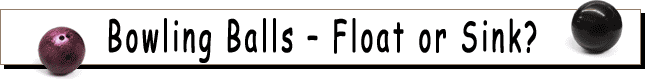We all know that heavy objects, such as a bowling ball will sink in water – right? Are you sure? Try this experiment to find out for yourself.

For this experiment you will need:

• a 11 pound or lighter bowling ball
• a 13 pound or heavier bowling ball
• a large aquarium, a bathtub, or a trash can
• a piece of string 30 inches or longer
• a ruler
• a bathroom scale

Fill the aquarium, bathtub, or trash can 3/4 of the way with water. Place the 13 pound or heavier bowling ball (CAREFULLY - DO NOT DROP) into the water. Does it sink or float? Is this what you expected? Now CAREFULLY place the 11 pound or lighter bowling ball into the water. Does it sink or float? Is this what you expected?

We can figure out if a bowling ball (or any object) will sink or float in water, if we know its density. Density is a measure of how much mass is present in a given volume. Once we know the density of an object, we can compare it to the density of water, which is about 1 g/cm3. If the density of the object is greater than the density of water, it will sink. If the density of the object is less than the density of water, it will float.

Let’s figure out the density of the bowling balls. To do this, we need to know their volume and their weight.

Measure the circumference of each of your bowling balls by wrapping a piece of string around the ball at its widest part, marking the circumference of the ball on the string. Next lay the string out flat and straight to measure the length with a ruler. What is the circumference of your ball? It should be pretty close to 27 inches. The rules of bowling state that "the circumference of a ball (the distance around it) shall not be more than 27.002 inches nor less than 26.704 inches”.

In science we usually measure using the metric system. You can convert inches to centimeters using the ratio 1 in. = 2.54 cm. What are the circumferences of the bowling balls in centimeters?

Now you can determine the volumes of your bowling balls using the equations for circumference and volume of a sphere. Remember that π is 3.14. The equation for circumference is:

circumference = 2 * π * radius

Once you have solved for the radius of each ball, you can find the volumes using the equation:

volume = 4/3 * π * radius3

You may notice that bowling balls are not perfect spheres. There are usually 3 or 4 holes in each ball. We must subtract the volume of these finger holes to determine the true volume of each ball. We can determine the volume of these holes by assuming they are roughly cylindrical in shape and measuring the depth and radius of the holes. An easy way to measure the depth of each of the finger holes is to put a pencil into the ball so that it touches the bottom of the finger hole. Mark the pencil at the point where it is even with the ball's surface by pinching the pencil between your fingers and lifting it out of the hole). Then measure the distance from your fingers to the end of the pencil. This is the depth of the finger hole. To find the volume you will need to also measure the radius of each hole (the radius is half the diameter or maximum width of the hole). Remember to convert your measurements to centimeters if you measured in inches. Now use the equation for the volume of a cylinder:

volume = π * radius2 * height

Determine the volume of each finger hole (are they different?) For each bowling ball, subtract the total volume of the finger holes from the volume of the sphere that you calculated earlier. This will give you more accurate values for the volumes of your bowling balls.

Now let’s find the weight of the balls using the bathroom scale. Some scales don’t measure small weights accurately, so you might try this technique: weigh yourself, then weigh yourself holding a bowling ball, and subtract the two weights to find the weight of the ball. Most scales in the U.S.give the weight in pounds. The conversion between pounds and grams is 1 lb = 453.6 g. Finally, for each ball divide the weight of your ball (in grams) by the volume of your ball in cubic centimeters to get their densities.

Compare the densities of the bowling balls to the density of water, which is around 1 g/cm3. If the density of a ball is less than 1 g/cm3, it will float. If it is greater than 1 g/cm3, it will sink. Do these results agree with what you saw when you put the balls in the water?

Did subtracting the volume of the finger holes affect your final volume calculation greatly? By what percentage did the volume of your ball change?

The density of steel is around 7.8 g/cm3, much greater than that of water. So how are large ships, which are made mostly of steel, able to stay afloat in water? It's important to keep in mind that the ability of an object to float is not entirely based on the density of the material that it is made out of. Ships are constructed in such a way that they contain a lot of open space inside them. The overall density of the ship includes all of the air inside it, so its average density is less than that of water.

Back to Home Experiments